# The Simplex Method[Page A-5 ( continued )]

The steps of the simplex method are carried out within the framework of a table, or tableau . The tableau organizes the model into a form that makes applying the mathematical steps easier. The Beaver Creek Pottery Company example will be used again to demonstrate the simplex tableau and method.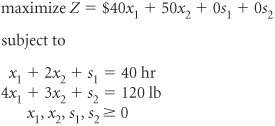The simplex method is a set of mathematical steps for solving a linear programming problem carried out in a table called a simplex tableau.

[Page A-6]

The initial simplex tableau for this model, with the various column and row headings, is shown in Table A-1.

##### Table A-1. The Simplex Tableau

Basic Variables

Quantity

c j

x 1

x 2

s 1

s 2

z j

c j z j

The first step in filling in Table A-1 is to record the model variables along the second row from the top. The two decision variables are listed first, in order of their subscript magnitude, followed by the slack variables, also listed in order of their subscript magnitude. This step produces the row with x 1 , x 2 , s 1 , and s 2 in Table A-1.

The next step is to determine a basic feasible solution. In other words, which two variables will form the basic feasible solution and which will be assigned a value of zero? Instead of arbitrarily selecting a point (as we did with points A, B, and C in the previous section), the simplex method selects the origin as the initial basic feasible solution because the values of the decision variables at the origin are always known in all linear programming problems. At that point x 1 = 0 and x 2 = 0; thus, the variables in the basic feasible solution are s 1 and s 2 .

 x 1 + 2 x 2 + s 1 = 04 0 + 2(0) + s 1 = 40 s 1 = 40 hr

and

 4 x 1 + 3 x 2 + s 2 = 120 4(0) + 3(0) + s 2 = 120 s 2 = 120 lb

The basic feasible solution in the initial simplex tableau is the origin where all decision variables equal zero.

In other words, at the origin, where there is no production, all resources are slack, or unused. The variables s 1 and s 2 , which form the initial basic feasible solution, are listed in Table A-2 under the column "Basic Variables," and their respective values, 40 and 120, are listed under the column "Quantity."

##### Table A-2. The Basic Feasible Solution

Basic Variables

Quantity

c j

x 1

x 2

s 1

s 2

s 1

40

s 2

120

z j

c j z j

At the initial basic feasible solution at the origin, only slack variables have a value greater than zero.

[Page A-7]

The initial simplex tableau always begins with the solution at the origin, where x 1 and x 2 equal zero. Thus, the basic variables at the origin are the slack variables, s 1 and s 2 . Since the quantity values in the initial solution always appear as the right-hand-side values of the constraint equations, they can be read directly from the original constraint equations.

The quantity column values are the solution values for the variables in the basic feasible solution.

The top two rows and bottom two rows are standard for all tableaus; however, the number of middle rows is equivalent to the number of constraints in the model. For example, this problem has two constraints; therefore, it has two middle rows corresponding to s 1 and s 2 . (Recall that n variables minus m constraints equals the number of variables in the problem with values of zero. This also means that the number of basic variables with values other than zero will be equal to m constraints.)

The number of rows in a tableau is equal to the number of constraints plus four.

Similarly, the three columns on the left side of the tableau are standard, and the remaining columns are equivalent to the number of variables. Since there are four variables in this model, there are four columns on the right of the tableau, corresponding to x 1 , x 2 , s 1 , and s 2 .

The number of columns in a tableau is equal to the number of variables (including slacks, etc.) plus three.

The next step is to fill in the c j values, which are the objective function coefficients, representing the contribution to profit (or cost) for each variable x j or s j in the objective function. Across the top row the c j values 40, 50, 0, and 0 are inserted for each variable in the model, as shown in Table A-3.

##### Table A-3. The Simplex Tableau with c j Values

Basic Variables

Quantity

40

50

c j

x 1

x 2

s 1

s 2

s 1

40

s 2

120

z j

c j z j

The c j values are the contribution to profit (or cost) for each variable.

The values for c j on the left side of the tableau are the contributions to profit of only those variables in the basic feasible solution, in this case s 1 and s 2 . These values are inserted at this location in the tableau so that they can be used later to compute the values in the z j row.

The columns under each variable (i.e., x 1 , x 2 , s 1 , and s 2 ) are filled in with the coefficients of the decision variables and slack variables in the model constraint equations. The s 1 row represents the first model constraint; thus, the coefficient for x 1 is 1, the coefficient for x 2 is 2, the coefficient for s 1 is 1, and the coefficient for s 2 is 0. The values in the s 2 row are the second constraint equation coefficients, 4, 3, 0, and 1, as shown in Table A-4.

##### Table A-4. The Simplex Tableau with Model Constraint Coefficients

Basic Variables

Quantity

40

50

c j

x 1

x 2

s 1

s 2

s 1

40

1

2

1

s 2

120

4

3

1

z j

c j z j

[Page A-8]

This completes the process of filling in the initial simplex tableau. The remaining values in the z j and c j z j rows, as well as subsequent tableau values, are computed mathematically using simplex formulas.

The following list summarizes the steps of the simplex method (for a maximization model) that have been presented so far.

1. First, transform all inequalities to equations by adding slack variables.

2. Develop a simplex tableau with the number of columns equaling the number of variables plus three, and the number of rows equaling the number of constraints plus four.

3. Set up table headings that list the model decision variables and slack variables.

4. Insert the initial basic feasible solution, which are the slack variables and their quantity values.

5. Assign c j values for the model variables in the top row and the basic feasible solution variables on the left side.

6. Insert the model constraint coefficients into the body of the table.

#### Computing the z j and c j z j Rows

So far the simplex tableau has been set up using values taken directly from the model. From this point on the values are determined by computation. First, the values in the z j row are computed by multiplying each c j column value (on the left side) by each column value under Quantity, x 1 , x 2 , s 1 , and s 2 and then summing each of these sets of values. The z j values are shown in Table A-5.

##### Table A-5. The Simplex Tableau with z j Row Values

Basic Variables

Quantity

40

50

c j

x 1

x 2

s 1

s 2

s 1

40

1

2

1

s 2

120

4

3

1

z j

c j c j

The z j row values are computed by multiplying the c j column values by the variable column values and summing.

For example, the value in the z j row under the quantity column is found as follows .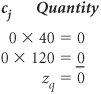The value in the z j row under the x 1 column is found similarly.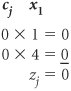All of the other z j row values for this tableau will be zero when they are computed using this formula.

The simplex method works by moving from one solution (extreme) point to an adjacent point until it locates the best solution.

Now the c j z j row is computed by subtracting the z j row values from the c j (top) row values. For example, in the x 1 column the c j z j row value is computed as 40 0 = 40. This value as well as other c j z j values are shown in Table A-6, which is the complete initial simplex tableau with all values filled in. This tableau represents the solution at the origin, where x 1 = 0, x 2 = 0, s 1 = 40, and s 2 = 120. The profit represented by this solution (i.e., the Z value) is given in the z j row under the quantity column0 in Table A-6. This solution is obviously not optimal because no profit is being made. Thus, we want to move to a solution point that will give a better solution. In other words, we want to produce either some bowls ( x 1 ) or some mugs ( x 2 ). One of the nonbasic variables (i.e., variables not in the present basic feasible solution) will enter the solution and become basic.

[Page A-9]

Basic Variables

Quantity

40

50

c j

x 1

x 2

s 1

s 2

s 1

40

1

2

1

s 2

120

4

3

1

z j

c j z j

40

50

#### The Entering Nonbasic Variable

As an example, suppose the pottery company decides to produce some bowls. With this decision x 1 will become a basic variable. For every unit of x 1 (i.e., each bowl) produced, profit will be increased by \$40 because that is the profit contribution of a bowl. However, when a bowl ( x 1 ) is produced, some previously unused resources will be used. For example, if

x 1 = 1

then

 x 1 + 2 x 2 + s 1 = 40 hr of labor 1 + 2(0) + s 1 = 40 s 1 = 39 hr of labor

and

 4 x 1 + 3 x 2 + s 2 = 120 lb of clay 4(1) + 3(0) + s 2 = 120 s 2 = 116 lb of clay

In the labor constraint we see that, with the production of one bowl, the amount of slack, or unused, labor is decreased by 1 hour . In the clay constraint the amount of slack is decreased by 4 pounds . Substituting these increases (for x 1 ) and decreases (for slack) into the objective function gives

 c j z j Z = 40(1) + 50(0) + 0(1) + 0(4) Z = \$40

The variable with the largest positive c j z j value is the entering variable.

The first part of this objective function relationship represents the values in the c j row; the second part represents the values in the z j row. The function expresses the fact that to produce some bowls, we must give up some of the profit already earned from the items they replace. In this case the production of bowls replaced only slack, so no profit was lost. In general, the c j z j row values represent the net increase per unit of entering a nonbasic variable into the basic solution . Naturally, we want to make as much money as possible, because the objective is to maximize profit. Therefore, we enter the variable that will give the greatest net increase in profit per unit. From Table A-7, we select variable x 2 as the entering basic variable because it has the greatest net increase in profit per unit, \$50 the highest positive value in the c j z j row.

[Page A-10]
##### Table A-7. Selection of the Entering Basic Variable

c j

Basic Variables

Quantity

40

50

x 1

x 2

s 1

s 2

s 1

40

1

2

1

s 2

120

4

3

1

z j

c j z j

40

50

The x 2 column, highlighted in Table A-7, is referred to as the pivot column . (The operations used to solve simultaneous equations are often referred to in mathematical terminology as pivot operations .)

The pivot column is the column corresponding to the entering variable.

The selection of the entering basic variable is also demonstrated by the graph in Figure A-2. At the origin nothing is produced. In the simplex method we move from one solution point to an adjacent point (i.e., one variable in the basic feasible solution is replaced with a variable that was previously zero). In Figure A-2 we can move along either the x 1 axis or the x 2 axis in order to seek a better solution. Because an increase in x 2 will result in a greater profit, we choose x 2 .

##### Figure A-2. Selection of which item to produce the entering basic variable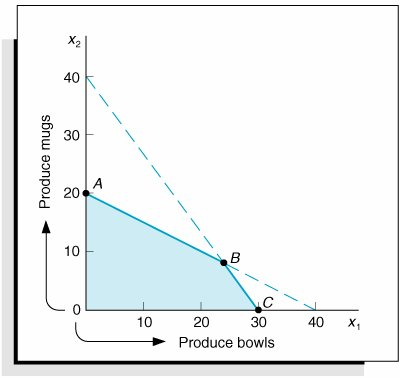#### The Leaving Basic Variable

Since each basic feasible solution contains only two variables with nonzero values, one of the two basic variables present, s 1 or s 2 , will have to leave the solution and become zero. Since we have decided to produce mugs ( x 2 ), we want to produce as many as possible or, in other words, as many as our resources will allow. First, in the labor constraint we will use all the labor to make mugs (because no bowls are to be produced, x 1 = 0; and because we will use all the labor possible and s 1 = unused labor resources, s 1 = 0 also).

[Page A-11]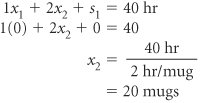In other words, enough labor is available to produce 20 mugs. Next, perform the same analysis on the constraint for clay.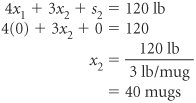This indicates that there is enough clay to produce 40 mugs. But there is enough labor to produce only 20 mugs. We are limited to the production of only 20 mugs because we do not have enough labor to produce any more than that. This analysis is shown graphically in Figure A-3.

##### Figure A-3. Determination of the basic feasible solution point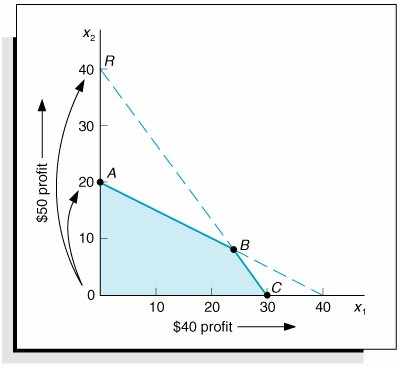Because we are moving out the x 2 axis, we can move from the origin to either point A or point R . We select point A because it is the most constrained and thus feasible, whereas point R is infeasible.

This analysis is performed in the simplex method by dividing the quantity values of the basic solution variables by the pivot column values. For this tableau,

Basic Variables

Quantity

x 2

s 1

40

· 2 = 20, the leaving basic variable

s 2

120

· 3 = 40

The leaving variable is determined by dividing the quantity values by the pivot column values and selecting the minimum possible value or zero .

[Page A-12]

The leaving basic variable is the variable that corresponds to the minimum nonnegative quotient, which in this case is 20. (Note that a value of zero would qualify as the minimum quotient and would be the choice for the leaving variable.) Therefore, s 1 is the leaving variable. (At point A in Figure A-3, s 1 equals zero because all the labor is used to make the 20 mugs.) The s 1 row, highlighted in Table A-8, is also referred to as the pivot row .

##### Table A-8. Pivot Column, Pivot Row, and Pivot Number

c j

Basic Variables

Quantity

40

50

x 1

x 2

s 1

s 2

s 1

40

1

2

1

s 2

120

4

3

1

z j

c j z j

40

50

The pivot row is the row corresponding to the leaving variable.

The value of 2 at the intersection of the pivot row and the pivot column is called the pivot number . The pivot number, row, and column are all instrumental in developing the next tableau. We are now ready to proceed to the second simplex tableau and a better solution.

The pivot number is the number at the intersection of the pivot column and row.

#### Developing a New Tableau

Table A-9 shows the second simplex tableau with the new basic feasible solution variables of x 2 and s 2 and their corresponding c j values.

##### Table A-9. The Basic Variables and c j Values for the Second Simplex Tableau

c j

Basic Variables

Quantity

40

50

x 1

x 2

s 1

s 2

50

x 2

s 2

z j

c j z j

The various row values in the second tableau are computed using several simplex formulas. First, the x 2 row, called the new tableau pivot row , is computed by dividing every value in the pivot row of the first (old) tableau by the pivot number. The formula for these computations is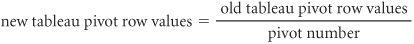Computing the new tableau pivot row values.

The new row values are shown in Table A-10.

##### (This item is displayed on page A-13 in the print version)

c j

Basic Variables

Quantity

40

50

x 1

x 2

s 1

s 2

50

x 2

20

1/2

1

1/2

s 2

z j

c j z j

To compute all remaining row values (in this case there is only one other row), another formula is used.

Computing all remaining row values.

[Page A-13]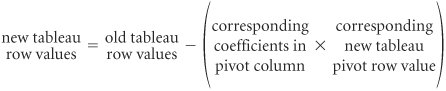Thus, this formula requires the use of both the old tableau and the new one. The s 2 row values are computed in Table A-11.

##### Table A-11. Computation of New s 2 Row Values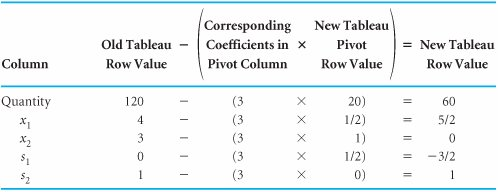These values have been inserted in the simplex tableau in Table A-12.

This solution corresponds to point A in the graph of this model in Figure A-3. The solution at this point is x 1 = 0, x 2 = 20, s 1 = 0, s 2 = 60. In other words, 20 mugs are produced and 60 pounds of clay are left unused. No bowls are produced and no labor hours remain unused.

##### Table A-12. The Second Simplex Tableau with Row Values

c j

Basic Variables

Quantity

40

50

x 1

x 2

s 1

s 2

50

x 2

20

1/2

1

1/2

s 2

60

5/2

3/2

1

z j

c j z j

The second simplex tableau is completed by computing the z j and c j z j row values the same way they were computed in the first tableau. The z j row is computed by summing the products of the c j column and all other column values.

[Page A-14]

## Time Out: For George B. Dantzig

After developing the simplex method for solving linear programming problems, George Dantzig needed a good problem to test it on. The problem he selected was the "diet problem" formulated in 1945 by Nobel economist George Stigler. This problem was to determine an adequate nutritional diet at minimum cost (which was an important military and civilian issue during World War II). Formulated as a linear programming model, the diet problem consisted of 77 unknowns and 9 equations. It took 9 clerks using hand-operated (mechanical) desk calculators 120 man-days to obtain the optimal simplex solution: a diet consisting primarily of wheat flour, cabbage, and dried navy beans that cost \$39.69 per year (in 1939 prices). The solution developed by Stigler using his own numerical method was only 24 cents more than the optimal solution.

 Column Quantity z j = (50) (20) + (0) (60) = 1000 x 1 z 1 = (50) (1/2) + (0) (5/2) = 25 x 2 z 2 = (50) (1) + (0) (0) = 50 s 1 z 3 = (50) (1/2) + (0) (3/2) = 25 s 2 z 4 = (50) (0) + (0) (1) = 0

The z j row values and the c j z j row values are added to the tableau to give the completed second simplex tableau shown in Table A-13. The value of 1,000 in the z j row is the value of the objective function (profit) for this basic feasible solution.

##### Table A-13. The Completed Second Simplex Tableau

c j

Basic Variables

Quantity

40

50

x 1

x 2

s 1

s 2

50

x 2

20

1/2

1

1/2

s 2

60

5/2

3/2

1

z j

1,000

25

50

25

c j z j

15

25

The computational steps that we followed to derive the second tableau in effect accomplish the same thing as row operations in the solution of simultaneous equations. These same steps are used to derive each subsequent tableau, called iterations .

Each tableau is the same as performing row operations for a set of simultaneous equations.

#### The Optimal Simplex Tableau

The steps that we followed to derive the second simplex tableau are repeated to develop the third tableau. First, the pivot column or entering basic variable is determined. Because 15 in the c j z j row represents the greatest positive net increase in profit, x 1 becomes the entering nonbasic variable. Dividing the pivot column values into the values in the quantity column indicates that s 2 is the leaving basic variable and corresponds to the pivot row. The pivot row, pivot column, and pivot number are indicated in Table A-14.

##### (This item is displayed on page A-15 in the print version)

Basic Variables

Quantity

40

50

c j

x 1

x 2

s 1

s 2

50

x 2

20

1/2

1

1/2

s 2

60

5/2

3/2

1

z j

1,000

25

50

25

c j z j

15

25

At this point you might be wondering why the net increase in profit per bowl ( x 1 ) is \$15 rather than the original profit of \$40. It is because the production of bowls ( x 1 ) will require some of the resources previously used to produce mugs ( x 2 ) only. Producing some bowls means not producing as many mugs; thus, we are giving up some of the profit gained from producing mugs to gain even more by producing bowls. This difference is the net increase of \$15.

[Page A-15]

The new tableau pivot row ( x 1 ) in the third simplex tableau is computed using the same formula used previously. Thus, all old pivot row values are divided through by 5/2, the pivot number. These values are shown in Table A-16. The values for the other row ( x 2 ) are computed as shown in Table A-15.

##### Table A-15. Computation of the x 2 Row for the Third Simplex Tableau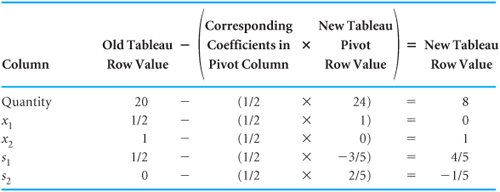##### Table A-16. The Completed Third Simplex Tableau

Basic Variables

Quantity

40

50

c j

x 1

x 2

s 1

s 2

50

x 2

8

1

4/5

1/5

40

x 1

24

1

3/5

2/5

z j

1,360

40

50

16

6

c j z j

16

6

These new row values, as well as the new z j row and c j z j row, are shown in the completed third simplex tableau in Table A-16.

Observing the c j z j row to determine the entering variable, we see that a nonbasic variable would not result in a positive net increase in profit, as all values in the c j z j row are zero or negative. This means that the optimal solution has been reached. The solution is

 x 1 = 24 bowls x 2 = 8 mugs Z = \$1,360 profit

The solution is optimal when all c j z j values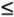0.

[Page A-16]

which corresponds to point B in Figure A-1.

An additional comment should be made regarding simplex solutions in general. Although this solution resulted in integer values for the variables (i.e., 24 and 8), it is possible to get a fractional solution for decision variables even though the variables reflect items that should be integers, such as airplanes, television sets, bowls, and mugs. To apply the simplex method, one must accept this limitation.

The simplex method does not guarantee integer solutions.Introduction to Management Science (10th Edition)
ISBN: 0136064361
EAN: 2147483647
Year: 2006
Pages: 358

Similar book on Amazon

flylib.com © 2008-2017.
If you may any questions please contact us: flylib@qtcs.net